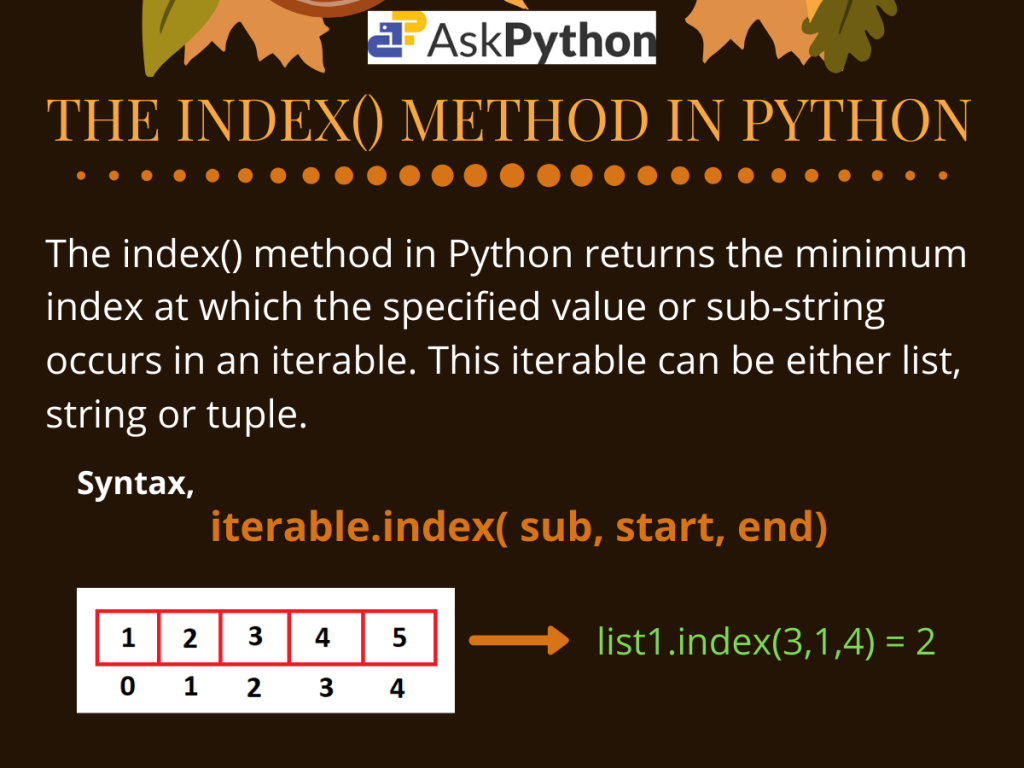# The Python index() Method## Introduction

So today in this tutorial, we are going to cover the Python index() method.

The `index()` method is defined for string, list and tuple class. For strings, the method returns the minimum index at which the given sub-string occurs. Whereas for lists and tuples, the method returns the minimum index where the given object if found.

## Using the Python index() Method

From the above definition, it is clear that the Python index() method is only defined for iterables. Hence, it would not work for dictionaries or sets as they don’t follow the indexing order.

For an iterable, the syntax for using the Python `index()` method is given below.

```iterable.index(sub, start, end)
```

Here,

• iterable can be any object like list, string or tuple,
• sub is the sub-string or item in the iterable whose minimum index is to be found,
• start is the starting index from where the search would start. It is set to 0 by default if not specified,
• end is the last index up to which the search would be done. Its value is considered equal to the length of the iterable if not specified.

Please note: If the object sub is not found within the range of start to end index, the method raises a `ValueError`.

## Examples for the index() Method in Python

Now that we know the syntax for using the `index()` method for any iterable, let us try to use it through some examples.

### 1. List index()

The `index()` method is a member function of the list class. And is widely used to search values in a list.

```# initialisation of variables
list1 = [9, 2, 7, 6, 8, 2, 3, 5, 1]

#index() with list
print("Value 2 first found at index: ", list1.index(2))
print("Value 2 first found at index(within range 4-7) : ", list1.index(2,4,7))
```

Output:

```Value 2 first found at index:  1
Value 2 first found at index(within range 4-7) :  5
```

Here in the above example, we have first initialized a list `list1`. Next, we try to get the minimum index where the value 2 occurs in it.

The program returns an index 1 when we try to find the value 2 with no start and end specified. Hence, it is clear that in the whole list, the `index()` method returns the minimum index where 2 is present.

Nextly for a specified range(4-7), the method gives us a value 5. This is the index where 2 occurs the second time within the list. But in the range 4-7, 5th index is the minimum one.

Note: the `index()` method works in the same manner for tuples too.

### 2. String Python index()

Coming to strings, the member function Python `index()` returns the minimum index at which the starting of the specified substring is found.

Let us look at an example.

```# initialisation of string
str1 = "Python Python"

#index() with string
print("sub-string 'Py' first found at index: ", str1.index('Py'))
print("sub-string 'Py' first found at index(within range 5-10) : ", str1.index('Py',5,10))
```

Output:

```sub-string 'Py' first found at index:  0
sub-string 'Py' first found at index(within range 5-10) :  7
```

Here for the first search with an unspecified range, the Python `index()` method returns 0 while searching the sub-string ‘Py‘. As we can see this is the minimum index at which ‘Py‘ occurs in the string `string1`.

When we specify a range(here 5-10) the method accordingly searches ‘Py’ from the 5th to 10th index. From the output, it is clear that the function finds the starting of the sub-string at 7th position.

## Conclusion

For any iterable it is worth noting that if the passed sub(object) is not found in the given iterable, a `ValueError` is raised.

So in this tutorial, we learned about the working as well as the use of the `index()` method in Python. For any further questions related to this topic, feel free to comment below.